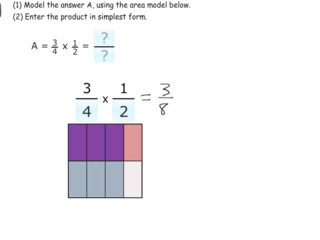# Interactive Problem Bank

•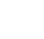Fraction Circles
•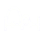Number Lines
•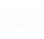Fraction Bars
•Sets
•Area Models
•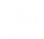Base Ten Blocks

Students multiply fractions using sets, fraction circles, rectangular models, and real-world contexts.

### Multiplication of Unit Fractions Using Number Line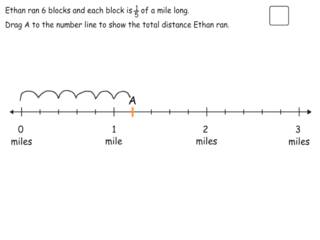### Multiplication of Fractions and Mixed Numbers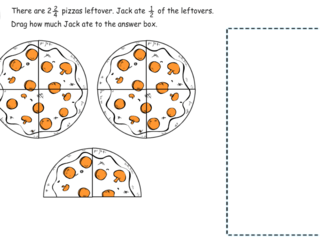### Multiplication of Fractions Using Models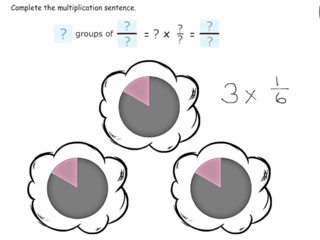### Multiplication of Whole Numbers by Mixed Numbers### Multiplication of Mixed Numbers by Whole Numbers Using Number Lines### Multiplication of a Fraction by a Fraction Using Area Model